#### 期刊菜单

Research on Control Strategy of Semi-Active Suspension Based on Random Road Surface Identification
DOI: 10.12677/DSC.2023.122013, PDF , HTML, XML, 下载: 118  浏览: 241

Abstract: To solve the time-delay problem of semi-active suspension system, an intelligent suspension control method based on the combination of random road surface identification and semi-active suspension control technology is proposed. At present, there are three modes of semi-active suspension system configured for mass production vehicles: comfort, standard and sport. Each mode is selected by the driver according to different road conditions, which leads to the limitation of semi- active suspension system, and cannot adaptively control the working conditions. In this paper, a convolutional neural network is constructed to classify and identify random road surfaces. The identified road roughness information is fed back to the semi-active suspension controller in advance, which can meet the adaptive suspension control under different road conditions. The effectiveness of the proposed algorithm is verified by vehicle road experiments.

1. 引言

2. 整车动力学模型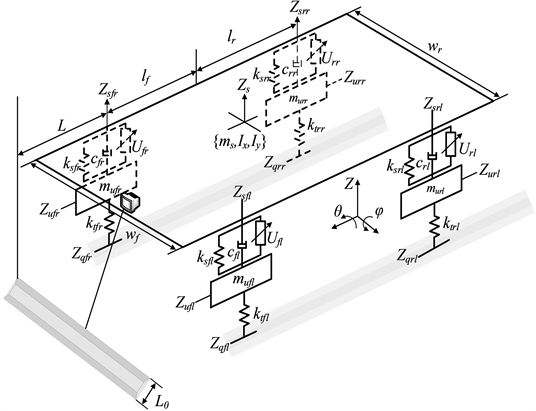Figure 1. Vehicle dynamics model

$\begin{array}{c}{m}_{s}{\stackrel{¨}{z}}_{s}={c}_{fl}\left({\stackrel{˙}{z}}_{ufl}-{\stackrel{˙}{z}}_{sfl}\right)+{k}_{sfl}\left(z{}_{ufl}-{z}_{sfl}\right)+{c}_{fr}\left({\stackrel{˙}{z}}_{ufr}-{\stackrel{˙}{z}}_{sfr}\right)\\ +{k}_{sfr}\left(z{}_{ufr}-{z}_{sfr}\right)+\text{\hspace{0.17em}}{c}_{rl}\left({\stackrel{˙}{z}}_{url}-{\stackrel{˙}{z}}_{srl}\right)+{k}_{srl}\left(z{}_{url}-{z}_{srl}\right)\\ +{c}_{rr}\left({\stackrel{˙}{z}}_{urr}-{\stackrel{˙}{z}}_{srr}\right)+{k}_{srr}\left(z{}_{urr}-{z}_{srr}\right)+{u}_{fl}+{u}_{fr}+{u}_{rl}+{u}_{rr}\end{array}$ (1)

$\begin{array}{c}{I}_{y}\stackrel{¨}{\varphi }={l}_{f}\left[{c}_{fl}\left({\stackrel{˙}{z}}_{ufl}-{\stackrel{˙}{z}}_{sfl}\right)+{k}_{sfl}\left({z}_{ufl}-{z}_{sfl}\right)\text{\hspace{0.17em}}+{c}_{fr}\left({\stackrel{˙}{z}}_{ufr}-{\stackrel{˙}{z}}_{sfr}\right)+\text{\hspace{0.17em}}{k}_{sfr}\left({z}_{ufr}-{z}_{sfr}\right)\right]\\ -l{}_{r}\left[{c}_{rl}\left({\stackrel{˙}{z}}_{url}-{\stackrel{˙}{z}}_{srl}\right)+{k}_{srl}\left({z}_{url}-{z}_{srl}\right)+\text{\hspace{0.17em}}{c}_{rr}\left({\stackrel{˙}{z}}_{urr}-{\stackrel{˙}{z}}_{srr}\right)+{k}_{srr}\left({z}_{urr}-{z}_{srr}\right)\right]\\ -{l}_{f}{u}_{fl}-{l}_{f}{u}_{fr}+{l}_{r}{u}_{rl}+{l}_{f}{u}_{rr}\end{array}$ (2)

$\begin{array}{c}{I}_{x}\stackrel{¨}{\theta }=\left[{c}_{fl}\left({\stackrel{˙}{z}}_{ufl}-{\stackrel{˙}{z}}_{sfl}\right)+{k}_{sfl}\left({z}_{ufl}-{z}_{sfl}\right)\text{\hspace{0.17em}}-{c}_{fr}\left({\stackrel{˙}{z}}_{ufr}-{\stackrel{˙}{z}}_{sfr}\right)-\text{\hspace{0.17em}}{k}_{sfr}\left({z}_{ufr}-{z}_{sfr}\right)\right]\frac{{w}_{f}}{2}\\ \text{+}\left[{c}_{rl}\left({\stackrel{˙}{z}}_{url}-{\stackrel{˙}{z}}_{srl}\right)+{k}_{srl}\left({z}_{url}-{z}_{srl}\right)\text{\hspace{0.17em}}-{c}_{rr}\left({\stackrel{˙}{z}}_{urr}-{\stackrel{˙}{z}}_{srrl}\right)+{k}_{srr}\left({z}_{urr}-{z}_{srr}\right)\right]\frac{{w}_{r}}{2}\\ -\frac{{w}_{f}}{2}{u}_{fl}+\frac{{w}_{f}}{2}{u}_{fr}-\frac{{w}_{r}}{2}{u}_{rl}+\frac{{w}_{r}}{2}{u}_{rr}\end{array}$ (3)

${m}_{ufl}{\stackrel{¨}{z}}_{ufl}={k}_{sfl}\left({z}_{sfl}-{z}_{ufl}\right)+{k}_{tfl}\left({z}_{qfl}-{z}_{ufl}\right)\text{\hspace{0.17em}}+{c}_{fl}\left({\stackrel{˙}{z}}_{sfl}-{\stackrel{˙}{z}}_{ufl}\right)-{u}_{fl}$ (4)

${m}_{ufr}{\stackrel{¨}{z}}_{ufr}={k}_{sfr}\left({z}_{sfr}-{z}_{ufr}\right)+{k}_{tfr}\left({z}_{qfr}-{z}_{ufr}\right)+{c}_{fr}\left({\stackrel{˙}{z}}_{sfr}-{\stackrel{˙}{z}}_{ufr}\right)-{u}_{fr}$ (5)

${m}_{url}{\stackrel{¨}{z}}_{url}={k}_{srl}\left({z}_{srl}-{z}_{url}\right)+{k}_{trl}\left({z}_{qrl}-{z}_{url}\right)+{c}_{rl}\left({\stackrel{˙}{z}}_{srl}-{\stackrel{˙}{z}}_{url}\right)-{u}_{rl}$ (6)

${m}_{urr}{\stackrel{¨}{z}}_{urr}={k}_{srr}\left({z}_{srr}-{z}_{urr}\right)+{k}_{trr}\left({z}_{qrr}-{z}_{urr}\right)+{c}_{rr}\left({\stackrel{˙}{z}}_{srr}-{\stackrel{˙}{z}}_{urr}\right)-{u}_{rr}$ (7)

$x={\left[{z}_{s}\text{\hspace{0.17em}}\theta \text{\hspace{0.17em}}\varphi \text{\hspace{0.17em}}{z}_{ufl}\text{\hspace{0.17em}}{z}_{ufr}\text{\hspace{0.17em}}{z}_{url}\text{\hspace{0.17em}}{z}_{urr}\text{\hspace{0.17em}}{\stackrel{˙}{z}}_{s}\text{\hspace{0.17em}}\stackrel{˙}{\theta }\text{\hspace{0.17em}}\stackrel{˙}{\varphi }\text{\hspace{0.17em}}\text{\hspace{0.17em}}{\stackrel{˙}{z}}_{ufl}\text{\hspace{0.17em}}{\stackrel{˙}{z}}_{ufr}\text{\hspace{0.17em}}{\stackrel{˙}{z}}_{url}\text{\hspace{0.17em}}{\stackrel{˙}{z}}_{urr}\right]}^{\text{T}}$ (8)

$w={\left[{z}_{qfl}\text{\hspace{0.17em}}{z}_{qfr}\text{\hspace{0.17em}}{z}_{qrl}\text{\hspace{0.17em}}{z}_{qrr}\right]}^{\text{T}}$ (9)

$u={\left[{u}_{fl}\text{\hspace{0.17em}}{u}_{fr}\text{\hspace{0.17em}}{u}_{rl}\text{\hspace{0.17em}}{u}_{rr}\right]}^{\text{T}}$ (10)

$\stackrel{˙}{x}=Ax+Bu+Ew$ (11)

3. 控制算法设计

3.1. 传统天棚阻尼控制算法

$\left\{\begin{array}{l}{C}_{sky}={C}_{\mathrm{max}}{\stackrel{˙}{x}}_{s}\left({\stackrel{˙}{x}}_{s}-{\stackrel{˙}{x}}_{u}\right)>0\\ {C}_{sky}={C}_{\mathrm{min}}{\stackrel{˙}{x}}_{s}\left({\stackrel{˙}{x}}_{s}-{\stackrel{˙}{x}}_{u}\right)\le 0\end{array}$ (12)

3.2. 混合自适应预瞄控制算法

${t}_{0}={L}_{0}/V\mathrm{cos}\chi \text{\hspace{0.17em}}\text{\hspace{0.17em}}$ (13)

$\tau =L/V$ (14)

$\tau -\epsilon \ge 0$ 时，自适应悬架控制的时间延迟将被预瞄控制完全抵消。以左前悬架为例，混合自适应悬架预瞄控制的动力学模型公式为

$\left\{\begin{array}{l}{m}_{sfl}{\stackrel{¨}{x}}_{sfl}+{k}_{sfl}\left({x}_{sfl}-{x}_{ufl}\right)+{C}_{sky（t+\tau ）}\left(\stackrel{˙}{x}{}_{sfl}-{\stackrel{˙}{x}}_{ufl}\right)=0\\ {m}_{ufl}{\stackrel{¨}{x}}_{ufl}+{C}_{sky\left(t+\tau \right)}\left({\stackrel{˙}{x}}_{ufl}-\stackrel{˙}{x}{}_{sfl}\right)+{k}_{sfl}\left({x}_{ufl}-{x}_{sfl}\right)+{k}_{tfl}\left({x}_{ufl}-{x}_{qfl\left(t+\tau \right)}\right)=0\end{array}\text{\hspace{0.17em}}$ (15)

$\left\{\begin{array}{l}\stackrel{˙}{x}={A}_{sp}x+{B}_{sp}{u}_{\left(t+\tau \right)}+{E}_{sp}w\\ y={C}_{sp}x+{D}_{sp}{u}_{\left(t+\tau \right)}\end{array}\text{\hspace{0.17em}}$ (16)

$\frac{{q}_{f}\left(s\right)}{{q}_{p}\left(s\right)}={\text{e}}^{-\tau s}$ (17)

$\varsigma \left(t\right)={A}_{\varsigma }\varsigma \left(t\right)+{B}_{\varsigma }{q}_{p}\left(t\right)$ (18)

$\varsigma =\left[\begin{array}{c}{\varsigma }_{1}\\ {\varsigma }_{2}\end{array}\right],\text{}{A}_{\varsigma }=\left[\begin{array}{cc}0& 1\\ {\alpha }_{0}& {\alpha }_{1}\end{array}\right],\text{}{B}_{\varsigma }=\left[\begin{array}{c}-2{\alpha }_{1}\\ 6{\alpha }_{0}\end{array}\right],\text{}{\alpha }_{0}=12/{\tau }^{2},\text{}{\alpha }_{1}=6/\tau$ (19)

$\left[\begin{array}{c}\stackrel{˙}{x}\\ \stackrel{˙}{\varsigma }\end{array}\right]=\left[\begin{array}{cc}A& E{D}_{\varsigma }\\ 0& {A}_{\varsigma }\end{array}\right]\left[\begin{array}{c}x\\ \varsigma \end{array}\right]+\left[\begin{array}{c}B\\ 0\end{array}\right]u+\left[\begin{array}{c}E{E}_{\varsigma }\\ {B}_{\varsigma }\end{array}\right]{q}_{p}$ (20)

${D}_{\varsigma }=\left[\begin{array}{c}0\\ 1\end{array}\right],{E}_{\varsigma }=\left[1\right]$ (21)

$C\left(t\right)=\left\{\begin{array}{l}{C}_{\mathrm{min}}\text{}\text{\hspace{0.17em}}路面等级\text{\hspace{0.17em}}“不严重\text{\hspace{0.17em}}”\\ {C}_{\mathrm{min}}+\delta {}_{1}C{}_{\mathrm{max}}\text{}\text{\hspace{0.17em}}路面等级\text{\hspace{0.17em}}“相对严重”\\ {C}_{\mathrm{max}}\text{}路面等级\text{\hspace{0.17em}}“严重\text{\hspace{0.17em}}”\end{array}$ (22)

4. 基于卷积神经网络的随机道路识别

4.1. VGG16网络

VGGNet网络使用33的卷积核的新型算子，通过不断的堆叠3 × 3卷积核以及使用2 × 2结构的最大池化层，建立了16层至19层较深的网络结构，提高了分类精度，但增加了处理时间，该网络具有结构简单、泛化性较好的优点，比较适用于图像特征提取及分类。本文首先选用的是具有16层深度的VGG16网络进行路面识别，其结构如图2所示。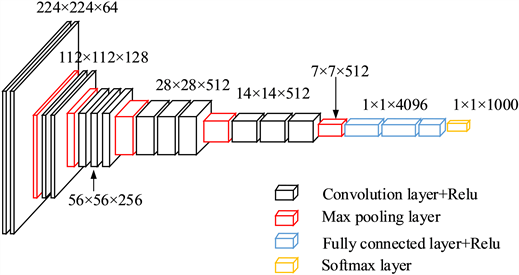Figure 2. VGG16 network architecture diagram

4.2. ResNet网络

ResNet网络在2015年ILSVRC分类比赛项目中取得了第一名的好成绩，其采用残差学习理论，使神经网络的卷积层扩展更深形成较深的网络结构，提升了目标分类的精度。本文选用的是具有50层深度的ResNet50网络模型对路面进行分类识别。残差网络可以直接将前一层的输出结果映射到后一层，并与后一层网络输出结果的差值作为新的学习目标，如图3所示，此种网络结构不再是完整的输出训练，而是采用残差方式保留了信息的完整性。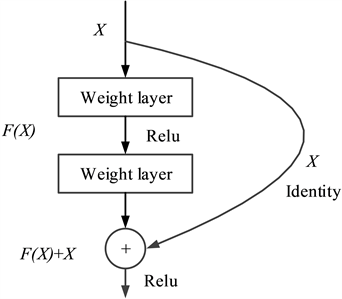Figure 3. ResNet residual learning module

4.3. 数据集选择

4.4. 实验结果分析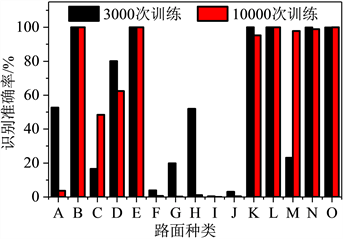Figure 4. VGG16 classification results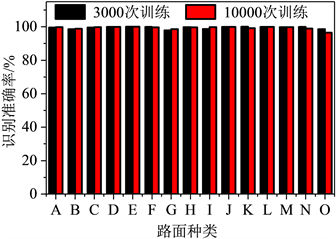Figure 5. ResNet classification results

5. 半主动悬架系统控制流程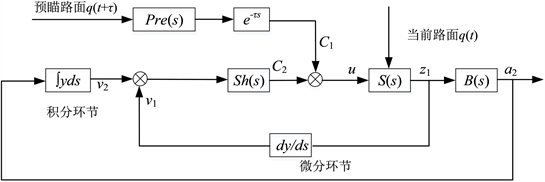Figure 6. Structure diagram of hybrid adaptive suspension preview control system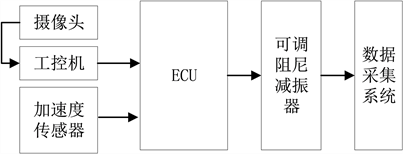Figure 7. System control flow chart

6. 整车道路实验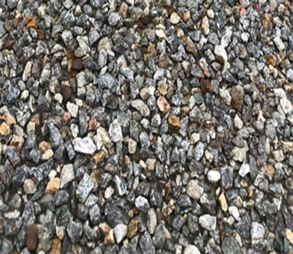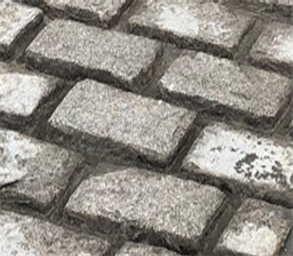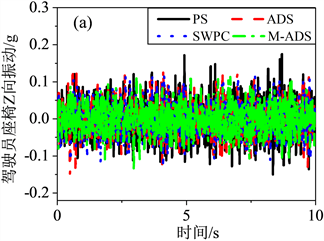Figure 10. Z-direction vibration data comparison of driver seat on 60 km/h rough asphalt road: (a) Time domain, (b) Frequency domain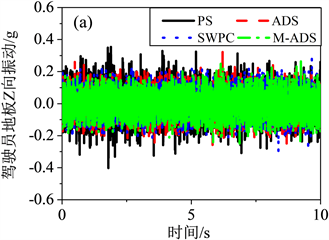Figure 11. Z-direction vibration data of driver floor of 60 km/h rough asphalt road: (a) Time domain, (b) Frequency domain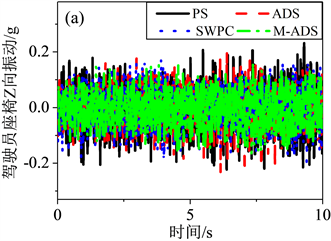Figure 12. Z-direction vibration data of driver seat at 40 km/h Belgium road: (a) Time domain, (b) Frequency domain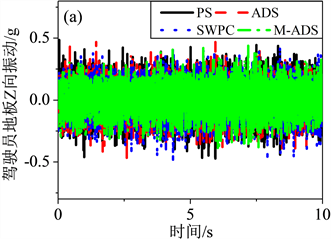Figure 13. Z-direction vibration data of driver floor of 40 km/h Belgium road: (a) Time domain, (b) Frequency domainTable 1. Test results under different control algorithms on random roads

7. 结论

1) 通过构建基于深度学习的VGG16和ResNet网络模型对随机路面进行识别并进行对比，ResNet模型的分类结果明显优于VGG16模型，主要是由于其采用残差学习理论，使神经网络的卷积层扩展更深形成较深的网络结构，保留了路面特征信息的完整性，大幅度提升了目标分类的精度。